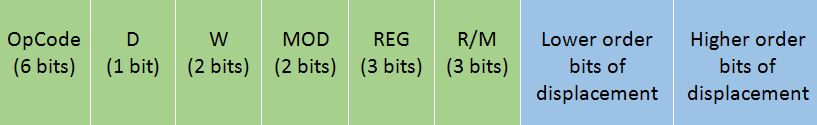# Instruction Format in 8086 Microprocessor

In this article, we are going to study the instruction format that is followed in the 8086 microprocessor.
Submitted by Monika Sharma, on July 22, 2019

For every instruction that is executed in the 8086 microprocessor, an instruction format is available that is the binary representation of that instruction.

This instruction format can be coded from 1 to 6 bytes depending upon the addressing modes used for instructions.

The general Instruction format that most of the instructions of the 8086 microprocessor follow is:• The Opcode stands for Operation Code. Every Instruction has a unique 6-bit opcode. For example, the opcode for MOV is 100010.
• D stands for direction
If D=0, then the direction is from the register
If D=1, then the direction is to the register
• W stands for word
If W=0, then only a byte is being transferred, i.e. 8 bits
If W=1, them a whole word is being transferred, i.e. 16 bits
• The MOD and R/M together is calculated based upon the addressing mode and register being used in it. This is calculated as follows:
• R/M 0 0
(Memory Mode with no displacement)
0 1
(Memory mode with 8 bit displacement)
1 0
(Memory Mode with 16 bit displacement)
1 1
(Register Mode)
000[BX] + [SI][BX] + [SI] + d8[BX] + [SI] + d16AL AX
001[BX] + [DI][BX] + [DI] + d8[BX] + [DI] + d16CL CX
010[BP] + [SI][BP] + [SI] + d8[BP] + [SI] + d16DL DX
011[BP] + [DI][BP] + [DI] + d8[BP] + [DI] + d16BL BX
100[SI][SI] + d8[SI] + d16AH SP
101[DI][DI] + d8[DI] + d16CH BP
110d16 (direct)[BP] + d8[BP] + d16DH SI
111[BX][BX] + d8[BX] + d16BH DI
• REG stands for register selected. It is a 3-bit code which is calculated as follows:
• REG Code Register Selected
0 0 0AL AX
0 0 1CL CX
0 1 0DL DX
0 1 1 BL BX
1 0 0AH SP
1 0 1CH BP
1 1 0DH SI
1 1 1 BH DI
• The low order displacement and high order displacement are optional and the instruction format contains them only if there exists any displacement in the instruction. If the displacement is of 8 bits, then only the cell of low order displacement infilled and if the displacement is of 16 bits, then both the cells od low order and high order are filled, with the exact bits that the displacement number represents.

What's New

Top Interview Coding Problems/Challenges!

Languages: » C » C++ » C++ STL » Java » Data Structure » C#.Net » Android » Kotlin » SQL
Web Technologies: » PHP » Python » JavaScript » CSS » Ajax » Node.js » Web programming/HTML
Solved programs: » C » C++ » DS » Java » C#
Aptitude que. & ans.: » C » C++ » Java » DBMS
Interview que. & ans.: » C » Embedded C » Java » SEO » HR
CS Subjects: » CS Basics » O.S. » Networks » DBMS » Embedded Systems » Cloud Computing
» Machine learning » CS Organizations » Linux » DOS
More: » Articles » Puzzles » News/Updates